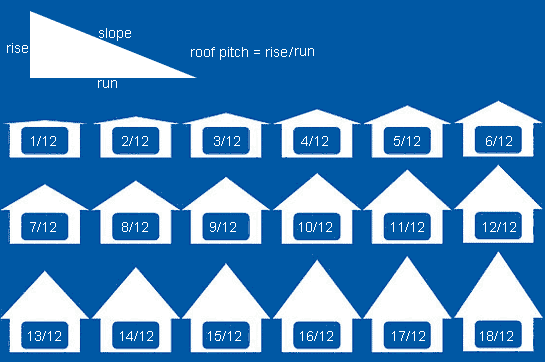# Determining Roof Pitch and Pitch Factor for Ordering Reflective InsulationUnlike traditional insulation that is installed in attic floors to keep heat inside buildings, many times radiant barriers and reflective insulation are installed at the roof line for the first line of defense to keep overhead heat out.

In residential construction, a radiant barrier traditionally is installed under the roof joists from inside the attic. For commercial or barn insulation, reflective insulation can be installed from the outside both over and under purlins or from the inside between the trusses below the purlins.

Simply taking the square footage (building width x length) is not enough to give an accurate material measurement. It is important in all roofline applications to understand the roof pitch and its corresponding pitch factor. Using these calculations will ensure you have enough material.

To determine roof pitch or slope we are back to mathematics and linear equations. Do you remember y = mx + b? Thankfully roof pitch can be derived another way. Regardless of what method you use some basic measurements are required. Remembering that slope or y = mx + b is equal to rise over run we must find two solid measuring points.

For true slop this would be best determined on an unfinished roof deck or atop the trusses. Shingles and metal roofing materials can change the measurements but for ordering insulation this degree of accuracy is not required.

To quickly determine rise, record the dimension from the top of the ridge down to bottom of the truss in a barn or commercial building. In a residential attic, record the measurement from the top of the ridge to the top of the floor joist or attic floor. To determine run, take the building width and divide by 2.We now have the rise and the run but there is one more step to determine pitch. In construction, the roof run is always measured by a unit of 12 so whatever the two measurements are the run needs to be expressed by 12.

Let's run through an example to demonstrate. We have a barn that we want to insulate and the measurement from the roof peak to the bottom of the truss is 12' and the building width is 36' wide. Following the dimensions above, we know the rise is 12 feet. To determine run, divide the building width by 2 which gives a dimension of 18'. We have determined the rise to be 12 and the run is 18. This is represented in a fraction.

12/18 can be expressed as 24/36 which can be expressed as 8/12. This building's roof has an 8/12 pitch. Notice the fraction is converted to express the run in a unit of 12.

Once the pitch is determined we use the chart to find the pitch factor. The pitch factor is the amount the square footage (width x length) needs to be multiplied by to take into account for the roof pitch. Let's use the same example from above and say the building is 60' long.

To get total square feet, multiply the width (36) x the length (60) = 2160. Looking at the pitch factor chart we see the factor for an 8/12 roof is 1.2. 2160 x 1.2 = 2,592.

2,592 square feet of insulation would be required to cover our example 36' x 60' barn with an 8/12 roof.